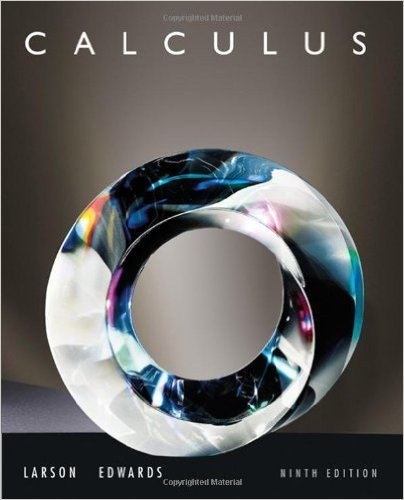×
×

# Solutions for Chapter 13.8: Extrema of Functions of Two Variables## Full solutions for Calculus | 9th Edition

ISBN: 9780547167022Solutions for Chapter 13.8: Extrema of Functions of Two Variables

Solutions for Chapter 13.8
4 5 0 253 Reviews
12
1
##### ISBN: 9780547167022

Calculus was written by and is associated to the ISBN: 9780547167022. This textbook survival guide was created for the textbook: Calculus , edition: 9. This expansive textbook survival guide covers the following chapters and their solutions. Since 64 problems in chapter 13.8: Extrema of Functions of Two Variables have been answered, more than 61157 students have viewed full step-by-step solutions from this chapter. Chapter 13.8: Extrema of Functions of Two Variables includes 64 full step-by-step solutions.

Key Calculus Terms and definitions covered in this textbook
• Arcsine function

See Inverse sine function.

• Basic logistic function

The function ƒ(x) = 1 / 1 + e-x

• Continuous function

A function that is continuous on its entire domain

• Coordinate(s) of a point

The number associated with a point on a number line, or the ordered pair associated with a point in the Cartesian coordinate plane, or the ordered triple associated with a point in the Cartesian three-dimensional space

• Degree

Unit of measurement (represented by the symbol ) for angles or arcs, equal to 1/360 of a complete revolution

• Differentiable at x = a

ƒ'(a) exists

• Equilibrium point

A point where the supply curve and demand curve intersect. The corresponding price is the equilibrium price.

• Future value of an annuity

The net amount of money returned from an annuity.

• General form (of a line)

Ax + By + C = 0, where A and B are not both zero.

• Hypotenuse

Side opposite the right angle in a right triangle.

• Inverse reflection principle

If the graph of a relation is reflected across the line y = x , the graph of the inverse relation results.

• Limaçon

A graph of a polar equation r = a b sin u or r = a b cos u with a > 0 b > 0

• Limit

limx:aƒ1x2 = L means that ƒ(x) gets arbitrarily close to L as x gets arbitrarily close (but not equal) to a

• Local extremum

A local maximum or a local minimum

• Product of a scalar and a vector

The product of scalar k and vector u = 8u1, u29 1or u = 8u1, u2, u392 is k.u = 8ku1, ku291or k # u = 8ku1, ku2, ku392,

• Real number line

A horizontal line that represents the set of real numbers.

• Sample space

Set of all possible outcomes of an experiment.

• System

A set of equations or inequalities.

• Term of a polynomial (function)

An expression of the form anxn in a polynomial (function).

• Xscl

The scale of the tick marks on the x-axis in a viewing window.

×